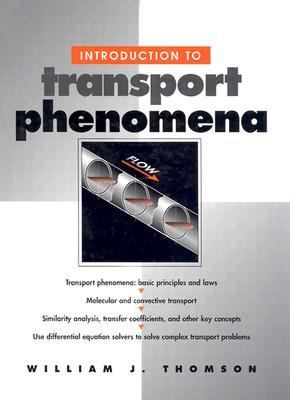216566

9780134548289

# Introduction to Transport PhenomenaOut of Stock

The item you're looking for is currently unavailable.

Most renters respond to questions in 48 hours or less.
The response will be emailed to you.
• ISBN-13: 9780134548289
• ISBN: 0134548280
• Publication Date: 2000
• Publisher: Prentice Hall

### AUTHOR

Thomson, William J.

### SUMMARY

45482-7 Transport phenomena: Fundamental concepts and problem solving Transport phenomena: basic principles and laws Molecular and convective transport Similarity analysis, transfer coefficients, and other key concepts Use differential equation solvers to solve complex transport problemsThis book is a true introduction to transport phenomena that presents all basic principles with a minimum of mathematical complexity. Readers will only need to know the basics of differential equations, and how to use a differential equation solver such as Matlab or ACSL.Professor William J. Thomson emphasizes the formulation of differential equations to describe physical problems, helping readers understand what they are doing-and why. The solutions are either simple (separable, linear second order) or derivable with a differential equation solver.Thomson begins with a detailed introduction to molecular transport, including the basic underlying laws, one-dimensional molecular energy transport, molecular mass and momentum transport principles, and transport coefficients. Each major similarity analysis technique is covered, including dimensionless groups in molecular transport, dimensionless differential transforms, and similarity transforms.In Part II, Thomson reviews convective transport, presenting a straightforward description of turbulence, and introducing the fundamental concept of transfer coefficients. Building on previous coverage, he then addresses the macroscopic calculation issues associated with momentum, heat, and mass transfer-enabling readers to solve even complex gas absorption and cooling tower problems.Thomson, William J. is the author of 'Introduction to Transport Phenomena', published 2000 under ISBN 9780134548289 and ISBN 0134548280.

You can find lots of answers to common customer questions in our FAQs

View a detailed breakdown of our shipping prices